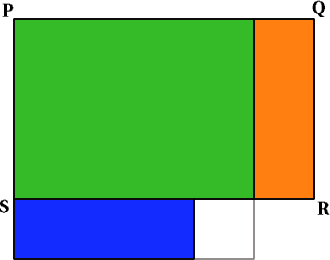#### You may also like### Diophantine N-tuples

Can you explain why a sequence of operations always gives you perfect squares?### DOTS Division

Take any pair of two digit numbers x=ab and y=cd where, without loss of generality, ab > cd . Form two 4 digit numbers r=abcd and s=cdab and calculate: {r^2 - s^2} /{x^2 - y^2}.### Sixational

The nth term of a sequence is given by the formula n^3 + 11n . Find the first four terms of the sequence given by this formula and the first term of the sequence which is bigger than one million. Prove that all terms of the sequence are divisible by 6.

# AMGM

##### Age 14 to 16Challenge LevelChoose any two numbers. Call them $a$ and $b$ ($b < a$). Work out the arithmetic mean $(a + b)/2$ and the geometric mean $\sqrt{ab}$. Which is bigger? Repeat for other pairs of numbers. What do you notice?

In the diagram PQRS is a rectangle measuring $a$ units by $b$ units. The green rectangle measures $(a + b)/2$ by $b$ and the orange and blue rectangles both measure $(a - b)/2$ by $b$. By considering the areas of the rectangles explain why this diagram shows that $$ab < ({{a + b}\over 2})^2.$$ What does this tell us about the arithmetic mean and the geometric mean of two numbers?